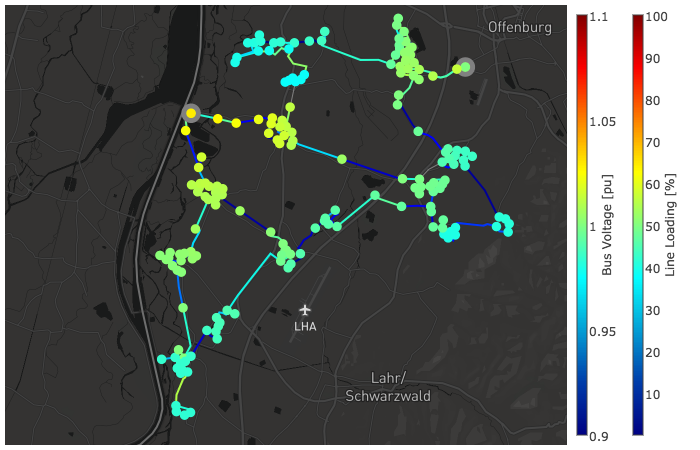Built-in plot functions¶

In order to get idea about interactive plot features and possibilities see the tutorial.

Simple Plotting¶

The function simple_plotly() can be used for a simple interactive plotting.

pandapower.plotting.plotly.simple_plotly(net, respect_switches=True, use_line_geodata=None, on_map=False, projection=None, map_style='basic', figsize=1, aspectratio='auto', line_width=1, bus_size=10, ext_grid_size=20.0, bus_color='blue', line_color='grey', trafo_color='green', ext_grid_color='yellow')

Plots a pandapower network as simple as possible in plotly. If no geodata is available, artificial geodata is generated. For advanced plotting see the tutorial

INPUT:

net - The pandapower format network. If none is provided, mv_oberrhein() will be plotted as an example

OPTIONAL:

respect_switches (bool, True) - Respect switches when artificial geodata is created

use_line_geodata* (bool, True) - defines if lines patches are based on net.line_geodata of the lines (True) or on net.bus_geodata of the connected buses (False)

on_map (bool, False) - enables using mapbox plot in plotly. If provided geodata are not real geo-coordinates in lon/lat form, on_map will be set to False.

projection (String, None) - defines a projection from which network geo-data will be transformed to lat-long. For each projection a string can be found at http://spatialreference.org/ref/epsg/

map_style (str, ‘basic’) - enables using mapbox plot in plotly

• ‘streets’

• ‘bright’

• ‘light’

• ‘dark’

• ‘satellite’

figsize (float, 1) - aspectratio is multiplied by it in order to get final image size

aspectratio (tuple, ‘auto’) - when ‘auto’ it preserves original aspect ratio of the network geodata any custom aspectration can be given as a tuple, e.g. (1.2, 1)

line_width (float, 1.0) - width of lines

bus_size (float, 10.0) - size of buses to plot.

ext_grid_size (float, 20.0) - size of ext_grids to plot.

See bus sizes for details. Note: ext_grids are plotted as rectangles

bus_color (String, “blue”) - Bus Color. Init as first value of color palette.

line_color (String, ‘grey’) - Line Color. Init is grey

trafo_color (String, ‘green’) - Trafo Color. Init is green

ext_grid_color (String, ‘yellow’) - External Grid Color. Init is yellow

Example plot with mv_oberrhein network from the pandapower.networks package: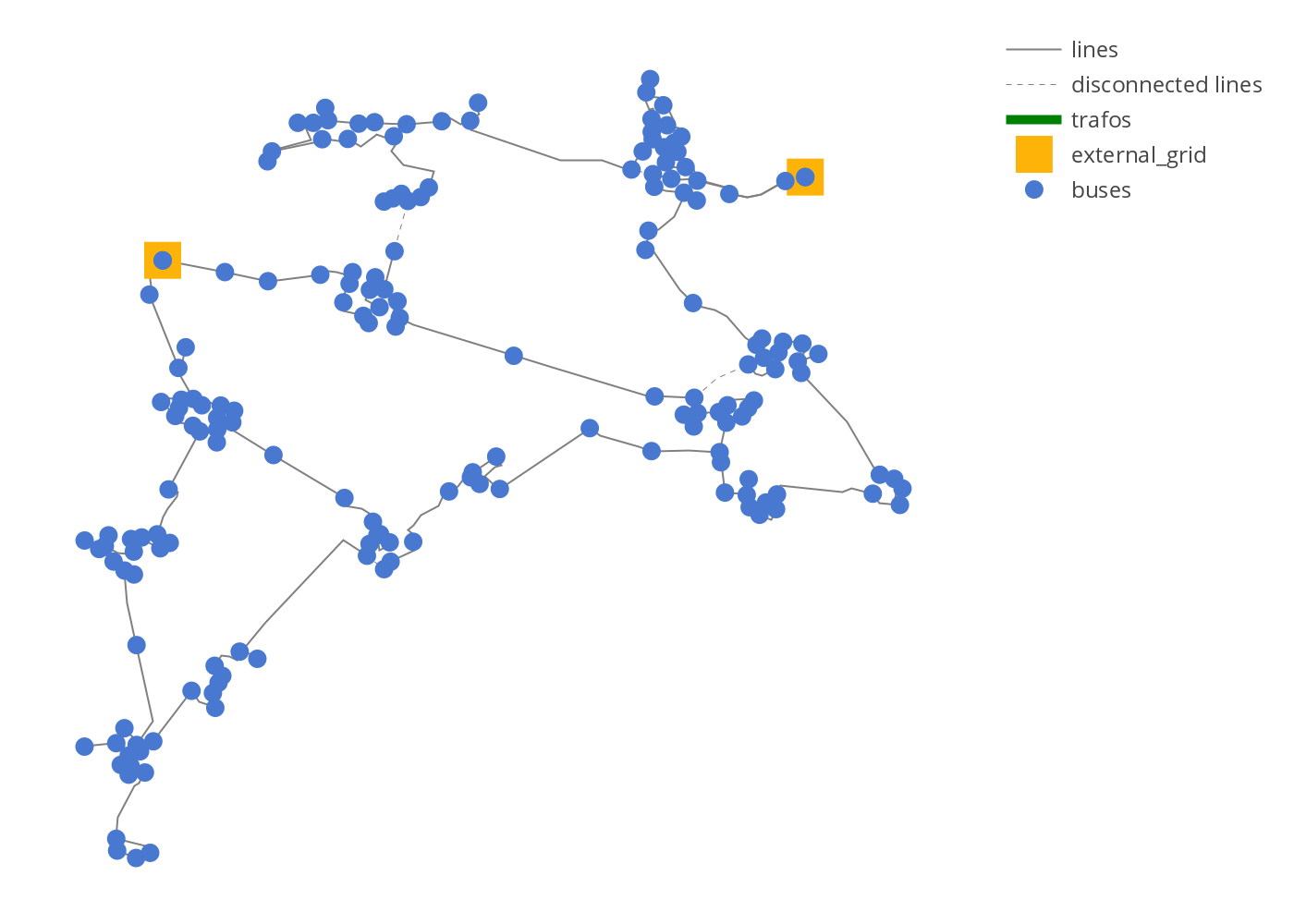Example simple plot

from pandapower.plotting.plotly import simple_plotly
from pandapower.networks import mv_oberrhein
net = mv_oberrhein()
simple_plotly(net)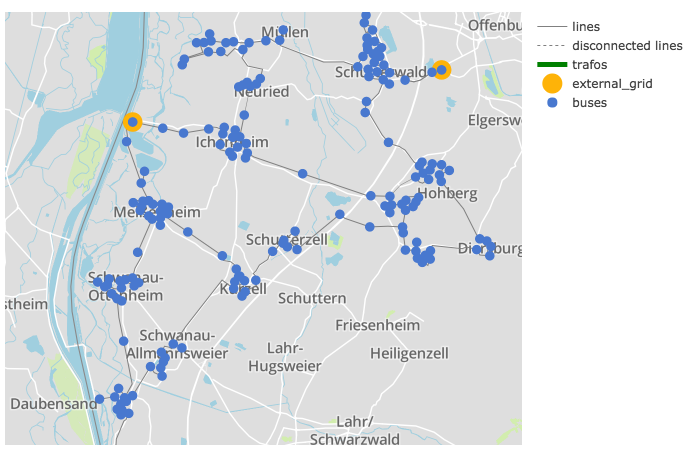Example simple plot on a map:

net = mv_oberrhein()
simple_plotly(net, on_map=True, projection='epsg:31467')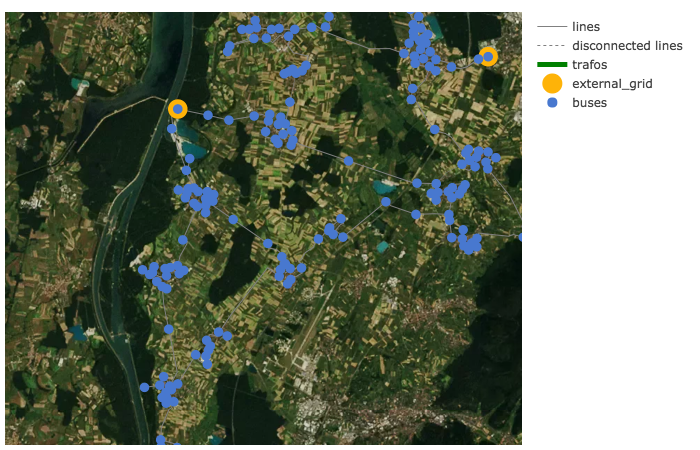Network coloring according to voltage levels¶

The function vlevel_plotly() is used to plot a network colored and labeled according to voltage levels.

pandapower.plotting.plotly.vlevel_plotly(net, respect_switches=True, use_line_geodata=None, colors_dict=None, on_map=False, projection=None, map_style='basic', figsize=1, aspectratio='auto', line_width=2, bus_size=10)

Plots a pandapower network in plotly using lines/buses colors according to the voltage level they belong to. If no geodata is available, artificial geodata is generated. For advanced plotting see the tutorial

INPUT:

net - The pandapower format network. If none is provided, mv_oberrhein() will be plotted as an example

OPTIONAL:

respect_switches (bool, True) - Respect switches when artificial geodata is created

use_line_geodata (bool, True) - defines if lines patches are based on net.line_geodata of the lines (True) or on net.bus_geodata of the connected buses (False)

colors_dict* (dict, None) - dictionary for customization of colors for each voltage level in the form: voltage_kv : color

on_map (bool, False) - enables using mapbox plot in plotly If provided geodata are not real geo-coordinates in lon/lat form, on_map will be set to False.

projection (String, None) - defines a projection from which network geo-data will be transformed to lat-long. For each projection a string can be found at http://spatialreference.org/ref/epsg/

map_style (str, ‘basic’) - enables using mapbox plot in plotly

• ‘streets’

• ‘bright’

• ‘light’

• ‘dark’

• ‘satellite’

figsize (float, 1) - aspectratio is multiplied by it in order to get final image size

aspectratio (tuple, ‘auto’) - when ‘auto’ it preserves original aspect ratio of the network geodata any custom aspectration can be given as a tuple, e.g. (1.2, 1)

line_width (float, 1.0) - width of lines

bus_size (float, 10.0) - size of buses to plot.

Example plot with mv_oberrhein network from the pandapower.networks package:

from pandapower.plotting.plotly import vlevel_plotly
from pandapower.networks import mv_oberrhein
net = mv_oberrhein()
vlevel_plotly(net)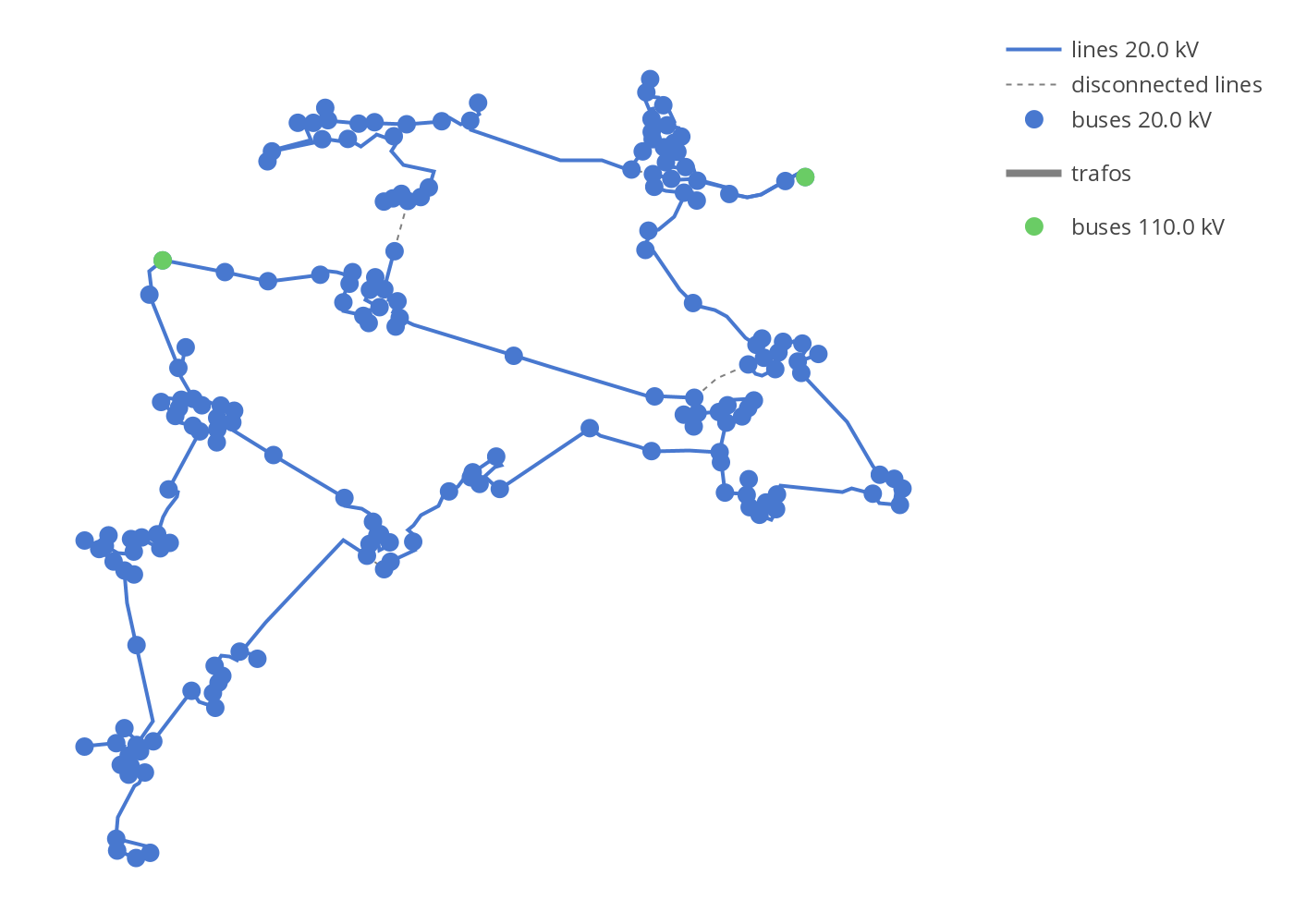Power Flow results¶

The function pf_res_plotly() is used to plot a network according to power flow results where a colormap is used to represent line loading and voltage magnitudes. For advanced possibilities see the tutorials.

pandapower.plotting.plotly.pf_res_plotly(net, cmap='Jet', use_line_geodata=None, on_map=False, projection=None, map_style='basic', figsize=1, aspectratio='auto', line_width=2, bus_size=10, filename='temp-plot.html')

Plots a pandapower network in plotly using colormap for coloring lines according to line loading and buses according to voltage in p.u. If no geodata is available, artificial geodata is generated. For advanced plotting see the tutorial

INPUT:

net - The pandapower format network. If none is provided, mv_oberrhein() will be plotted as an example

OPTIONAL:

respect_switches (bool, False) - Respect switches when artificial geodata is created

cmap* (str, True) - name of the colormap

colors_dict* (dict, None) - by default 6 basic colors from default collor palette is used. Otherwise, user can define a dictionary in the form: voltage_kv : color

on_map (bool, False) - enables using mapbox plot in plotly If provided geodata are not real geo-coordinates in lon/lat form, on_map will be set to False.

projection (String, None) - defines a projection from which network geo-data will be transformed to lat-long. For each projection a string can be found at http://spatialreference.org/ref/epsg/

map_style (str, ‘basic’) - enables using mapbox plot in plotly

• ‘streets’

• ‘bright’

• ‘light’

• ‘dark’

• ‘satellite’

figsize (float, 1) - aspectratio is multiplied by it in order to get final image size

aspectratio (tuple, ‘auto’) - when ‘auto’ it preserves original aspect ratio of the network geodata any custom aspectration can be given as a tuple, e.g. (1.2, 1)

line_width (float, 1.0) - width of lines

bus_size (float, 10.0) - size of buses to plot.

filename (str, “temp-plot.html”) - filename / path to plot to. Should end on *.html

Example power flow results plot:

from pandapower.plotting.plotly import pf_res_plotly
from pandapower.networks import mv_oberrhein
net = mv_oberrhein()
pf_res_plotly(net)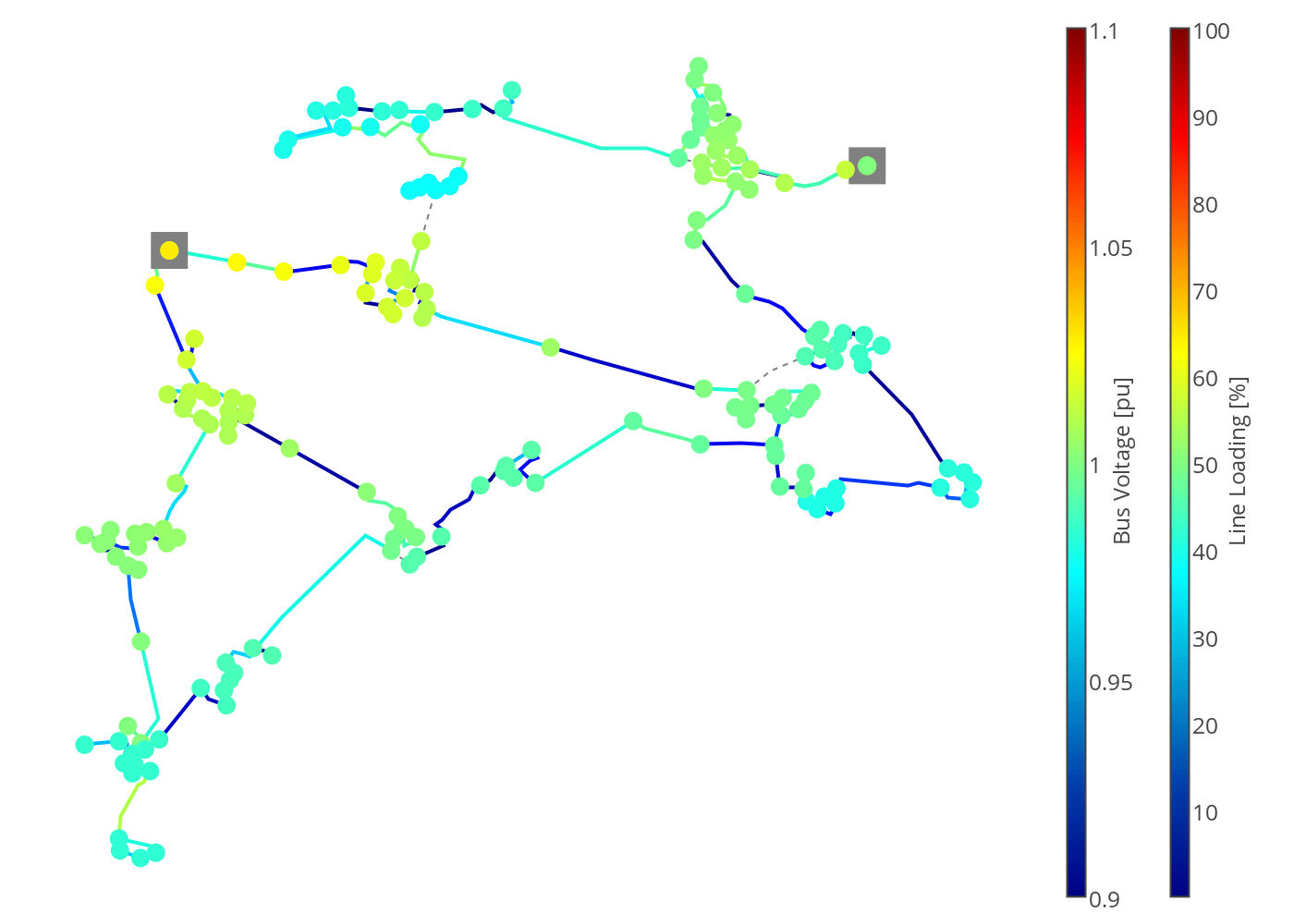Power flow results on a map:

net = mv_oberrhein()
pf_res_plotly(net, on_map=True, projection='epsg:31467', map_style='dark')